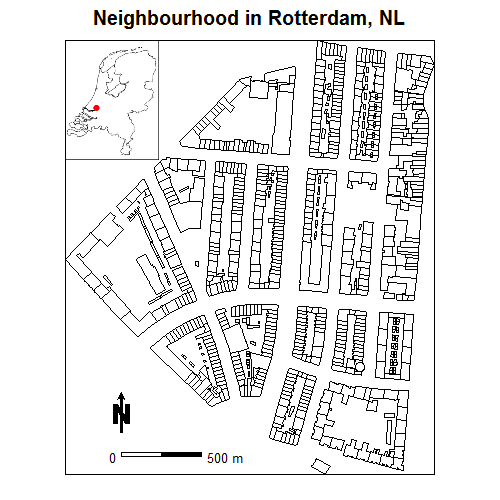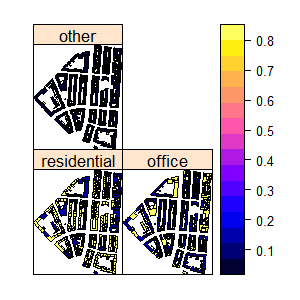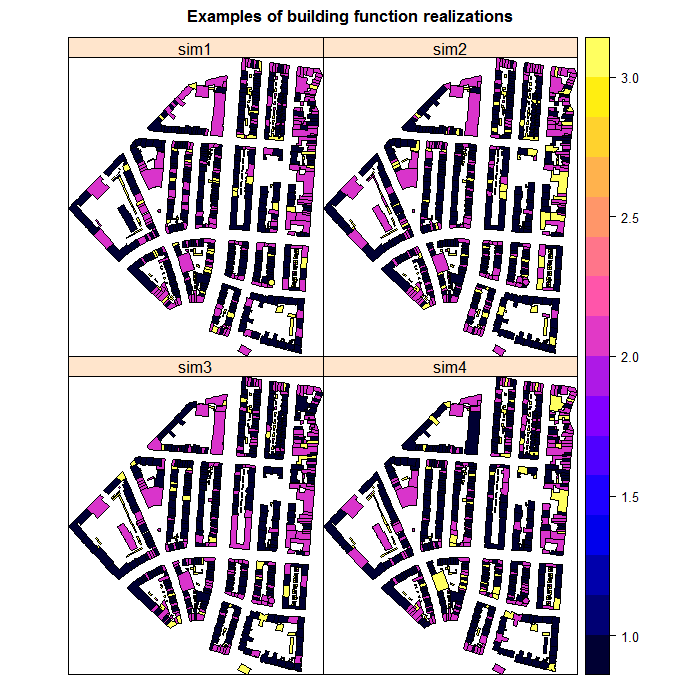Sys.sleep(100)

Case study with categorical data - calculating tax depending on a building function

Introduction

In many aspects of life information is available and required in a form of categorical data. For example, in a city neighbourhood or a district each building has assigned a function (housing, office, etc.). Depending on the building function the city council may impose different tax levels.

In this simple example, in a neighbourhood the function of a building is assigned depending on the building percentage of area inside the building that is used for living and number of addresses inside each building. If the residential area is higher than 80% and has at least one address registered then the building is classified as “residential”. If the residential area is less than 80% and at least one address is present the building is classified as “office”. If no address is present the building function is assumed as “other”. Depending of these categories the city council imposes tax levels of €1000, €10000, €10 per year, respectively. The 80% threshold is approximate though and some buildings classified as “others” could have got the address assigned but not put on a system yet, therefore the council wants to calculate the uncertainty in tax revenue depending on errors introduced by erroneous building classification.

Adapted Monte Carlo methodology for spatial uncertainty analysis with a categorical variable

The adapted uncertainty propagation analysis approach is based on the Monte Carlo method that computes the output of the model repeatedly, with input values that are randomly sampled from their non-parameteric pdfs. The set of model outputs forms a random sample from the output pdf. The method thus consists of the following steps:

1. Characterise uncertain model inputs with non-parametric pdfs (i.e. probabilities for each category).
2. Repeatedly sample from the pdfs of uncertain inputs.
3. Run model with sampled inputs and store model outputs.
4. Compute summary statistics of model outputs.

Building function uncertainty propagation analysis with ‘spup’

Preliminaries - load and view the data

The example data for tax calculation contain the spatial distribution of buildings in a neighbourhood in Rotterdam, NL.

library(sp)
library(spup)
library(purrr)
library(png)

set.seed(12345)

data(woon)
# Netherlands contour and Rtterdam location
# collate info about fugure size and type
NL_map <- list("grid.raster", NL, x = 89987, y = 436047, width = 120,
height = 152, default.units = 'native')
# collate info about a scale bar location in the figure, type and colour
scale <- list("SpatialPolygonsRescale", layout.scale.bar(),
offset = c(90000,435600), scale = 100, fill=c("transparent","black"))
# collate info about minimum value on a scale bar
text1 <- list("sp.text", c(89990,435600), "0", cex = 0.8)
# collate info about maximum value on a scale bar
text2 <- list("sp.text", c(90130,435600), "500 m", cex = 0.8)
# collate info about North arrow
arrow <- list("SpatialPolygonsRescale", layout.north.arrow(),
offset = c(89990,435630), scale = 50)
# plot 'woon' object with a location miniature, North arrow and scale bar defined above
spplot(woon, "check", do.log = TRUE, col.regions = "transparent",
colorkey = FALSE, key.space = list(x = 0.1, y = 0.93, corner = c(0,1)),
sp.layout = list(scale, text1, text2 ,arrow, NL_map),
main = "Neighbourhood in Rotterdam, NL")The ‘woon’ object is a SpatialPolygonDataFrame where each building is represented by one polygon. The attributes contain:

• vbos - number of addresses present in the building,
• woonareash - residential area [%],
• Function - assigned category depending on vbos and woonareash - for residential it is 1, for office it is 2, for other is 3,
• residential - probability that the building is residential,
• office - probability that the building is an office,
• other - probability that the building has another function.

In the next section we explain how we use the information about the probabilities.

Define uncertainty model (UM) for the building function

In case of categorical variables the uncertainty is described by use of non-parameteric pdfs. In our case we describe it by a vector of probabilities that a building belongs to a certain category. In case of spatially distributed variables we have to do this for each polygon, hence the dataset has maps of these probabilities saved in the same object.

To unite all information of the uncertainty model for the building function we use the defineUM() function that collates all information into one object. The minimum information required is:

• a logical value that indicates if the object is uncertain,
• a vector of categories; character and numeric type is allowed,
• a data frame with probabilities for each category.
# define uncertainty model for the bulding function
woonUM <- defineUM(TRUE, categories = c(1,2,3), cat_prob = woon[, c(4:6)])
class(woonUM)
 "MarginalCategoricalSpatial"

The argument cat_prob is the probabilities vector. In case of spatial vatiables for each polygon a vector of probabilities is collated into a data frame (as above). Foreach polygon, the probabilities vector should sum to one:

woon$check <- woon$residential + woon$office + woon$other
summary(woon$check) Min. 1st Qu. Median Mean 3rd Qu. Max. 1 1 1 1 1 1 We can have a look at how the probabilities for each polygon look like: spplot(woon[c(4,5,6)])Generate possible realities of the building function Generating possible realities of the building function can be completed by using the genSample() function. The required information to pass to the function includes: • defined uncertain object (as above). • number of realizations to return. Usually the sample must be large to obtain stable results. Let us run the sampling to obtain 10 realizations. Note the artument ‘asList’ has been set up to FALSE. This indicates that the sampling function will return an object of a class of the distribution parameters class. This is useful if you want to visualize the sample or compute summary statistics quickly. # create possible realizations of the building function woon_sample <- genSample(woonUM, 10, asList = FALSE) class(woon_sample)  "SpatialPolygonsDataFrame" attr(,"package")  "sp" # view several realizations spplot(woon_sample[c(3,4,1,2)], main = list(label = "Examples of building function realizations", cex = 1))Uncertainty propagation through the model that calculates tax revenue for the neighbourhood In order to perform uncertainty propagation analysis using ‘spup’, the model through which uncertainty is propagated needs to be defined as an R function, for example: tax <- function(building_Function) { building_Function$tax2pay <- NA
building_Function$tax2pay[building_Function$Function == 1] <- 1000
building_Function$tax2pay[building_Function$Function == 2] <- 10000
building_Function$tax2pay[building_Function$Function == 3] <- 10
total_tax <- sum(building_Function\$tax2pay)
total_tax
}

Note that in a given the model has a requirement that the building function is stored in a SpatialPolygonDataFrame and in a column named ‘Function’.

The propagation of uncertainty occurs when the model is run with an uncertain input. Running the model with a sample of realizations of uncertain input variable(s) yields an equally large sample of model outputs that can be further analyzed. To run the tax model with the building function realizations we use the propagate() function. The propagate() function takes as arguments:

• a sample from the uncertain model inputs and any other remaining model inputs and parameters as a list.
• the model as a function in R.
• the number of Monte Carlo runs. This can be equal or smaller than the number of realizations of the uncertain input variable(s).

In order to run the propagation function the sample of an uncertain input variable must be saved in a list. We can either coerce the existing woon_sample object or get it automatically setting up the ‘asList’ argument of genSample() to TRUE.

# coerce  SpatialPolygonDataFrame to a list of individual SpatialPolygonDataFrames
woon_sample <- map(1:ncol(woon_sample), function(x){woon_sample[x]})

# or sample from uncertain input and save it in a list
woon_sample <- genSample(UMobject = woonUM, n = 10, asList = TRUE)
class(woon_sample)
 "list"

Earlier we noted that the construction of the model requires that the information of the building function is stored in a column named ‘Function’. By default the sampling function returns ralizations named ‘sim1’, ‘sim2’, and so on, so we need to adjust that manualy.

for (i in 1:10) names(woon_sample[[i]]) <- "Function"

Finally, run the propagation:

# run uncertainty propagation
tax_sample <- propagate(woon_sample, model = tax, n = 10)
tax_sample
[]
 2227530

[]
 2355420

[]
 2197560

[]
 2433360

[]
 2334450

[]
 2378370

[]
 2361360

[]
 2365410

[]
 2336610

[]
 2194680
summary(unlist(tax_sample))
Min. 1st Qu.  Median    Mean 3rd Qu.    Max.
2194680 2254260 2346015 2318475 2364398 2433360

We can see that on average the city council should obtain the tax revenue of €2300000, but depending on the building classification this amount may vary by €120000.

Acknowledgements

The Rotterdam neighbourhood dataset was obtained from the Dutch cadastral dataset BAG. We would like to thank Filip Biljecki for help with obtaining the data.

This project has received funding from the European Union’s Seventh Framework Programme for research, technological development and demonstration under grant agreement no 607000.